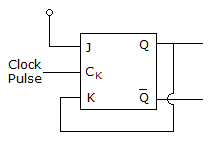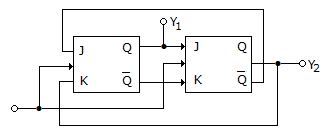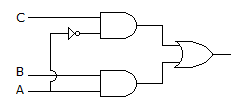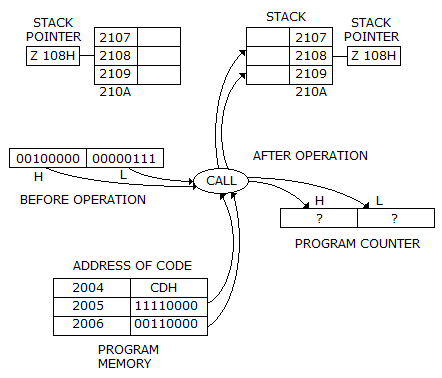# Test: Digital Electronics - 1

## 25 Questions MCQ Test Digital Electronics | Test: Digital Electronics - 1

Description
This mock test of Test: Digital Electronics - 1 for Electrical Engineering (EE) helps you for every Electrical Engineering (EE) entrance exam. This contains 25 Multiple Choice Questions for Electrical Engineering (EE) Test: Digital Electronics - 1 (mcq) to study with solutions a complete question bank. The solved questions answers in this Test: Digital Electronics - 1 quiz give you a good mix of easy questions and tough questions. Electrical Engineering (EE) students definitely take this Test: Digital Electronics - 1 exercise for a better result in the exam. You can find other Test: Digital Electronics - 1 extra questions, long questions & short questions for Electrical Engineering (EE) on EduRev as well by searching above.
QUESTION: 1

Solution:
QUESTION: 2

Solution:
QUESTION: 3

### In the given figure assume that initially Q = 1 with Clock Pulses being given, the subsequent states of Q will beSolution:
QUESTION: 4

If Vin is 0.99 V, what is the digital output of the ADC0801 after INTER goes low?

Solution:
QUESTION: 5

Which of the following operation are performed on linear queues?

1. Testing a linear queue for underflow
2. Enqueue operation
3. Dequeue operation
4. Testing a linear queue for overflow.
Select the correct answer using the codes given below the lists
Solution:
QUESTION: 6

As access time is decreased, the cost of memory

Solution:
QUESTION: 7

A 2 bit binary multiplier can be implemented using

Solution:
QUESTION: 8

Assertion (A): Hamming code is commonly used for error correction

Reason (R): In Hamming code the number of parity bits increases as the number of information bits increases.

Solution:
QUESTION: 9

A microcomputer has memory locations from 0000 to FFFF, each storing 1 byte. How many bytes can be the memory store?

Solution:
QUESTION: 10

Typical size of digital IC is about

Solution:
QUESTION: 11

In 8085, usually the vector location and the next two memory location contain a JMP instruction. This allows the programs to branch to

Solution:
QUESTION: 12

A clock signal coordinates the working of different flip flops.

Solution:
QUESTION: 13

The rate of change of digital signals between High and Low level is

Solution:
QUESTION: 14

In a positive-edge-triggered JK flip-flop, a low J and a low K produce __________ state. A high __________ on the rising edge of the clock.

Solution:
QUESTION: 15

In which of these does thermionic emission occur?

Solution:
QUESTION: 16

Find the decimal value of 111001:

Solution:
QUESTION: 17

Which of the following is best suited for parity checking and parity generation?

Solution:
QUESTION: 18

In the circuit shown below, the outputs Y1 and Y2 for the given initial condition Y1 = Y2 = 1 and after four input pulses will beSolution:
QUESTION: 19

The op amp is used in

Solution:
QUESTION: 20

Assertion (A): Power drain of CMOS increases with operating frequency

Reason (R): All unused CMOS inputs should be tied either to a fixed voltage level (0 or VDD) or to another input.

Solution:
QUESTION: 21

For the logic circuit of the given figure the simplified Boolean expression isSolution:
QUESTION: 22

In two level logic, logic race does not occur.

Solution:
QUESTION: 23

A digital clock uses __________ chip

Solution:
QUESTION: 24

Which of the following conversion is incorrect?

Solution:
QUESTION: 25

The contents of stack location 2109 H after the call operation will beSolution: